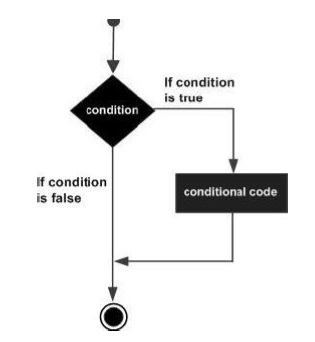# ES6 - if Statement

The ‘if…else’ construct evaluates a condition before a block of code is executed.

Following is the syntax.

```if(boolean_expression) {
// statement(s) will execute if the Boolean expression is true
}
```

If the Boolean expression evaluates to true, then the block of code inside the if statement will be executed. If the Boolean expression evaluates to false, then the first set of code after the end of the if statement (after the closing curly brace) will be executed.

## Flowchart## Example

```var num = 5
if (num>0) {
console.log("number is positive")
}
```

The following output is displayed on successful execution of the above code.

```number is positive
```

The above example will print “number is positive” as the condition specified by the if block is true.